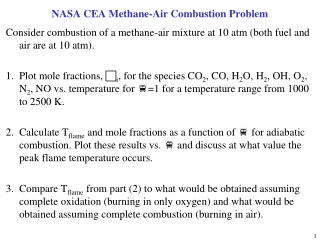DownloadDownload PresentationNASA CEA Methane-Air Combustion Problem

# NASA CEA Methane-Air Combustion Problem

Télécharger la présentation## NASA CEA Methane-Air Combustion Problem

- - - - - - - - - - - - - - - - - - - - - - - - - - - E N D - - - - - - - - - - - - - - - - - - - - - - - - - - -
##### Presentation Transcript

1. NASA CEA Methane-Air Combustion Problem Consider combustion of a methane-air mixture at 10 atm (both fuel and air are at 10 atm). • Plot mole fractions, ci, for the species CO2, CO, H2O, H2, OH, O2, N2, NO vs. temperature for f=1 for a temperature range from 1000 to 2500 K. • Calculate Tflame and mole fractions as a function of f for adiabatic combustion. Plot these results vs. f and discuss at what value the peak flame temperature occurs. • Compare Tflame from part (2) to what would be obtained assuming complete oxidation (burning in only oxygen) and what would be obtained assuming complete combustion (burning in air).

2. Turbofan Problem • In some applications of turbofan engines it is desirable to mix the core and fan airflows, either before they enter an afterburner or before they exit a common nozzle. • Such mixing schemes have been used in low-bypass engines for both commercial transports and for military fighter applications. • In such cases it is reasonable to set the fan discharge stagnation pressure equal to that at the exit form the turbine. Note that this is not always the best choice, but a reasonable first approximation to modeling requirement that the two streams must mix without large losses. • Develop an expression for the thrust of such a mixed-flow turbofan by imposing the condition that the stagnation pressures at the fan exit and the turbine exit must be equal. Note that this condition replaces the condition that the exhaust velocities be equal in setting the fan pressure ratio. Keep the bypass ratio as a parameter, and let the compressor pressure ratio be that for maximum (non-afterburning thrust). • Compare the thrust of this engine with that of an engine (of the same bypass ratio) but with equal core and fan jet velocities.

3. Non-ideal Inlet Behavior • In all of the discussions that we have had in class, we have assumed that the compression in the inlet is reversible and adiabatic. To see how restrictive this assumption is: • Re-derive the expression for the thrust of the turbojet engine, including the possibility of stagnation pressure losses in the inlet. Assume that the rest of components are ideal. • Write an expression for the T/A2P0, accounting for total pressure loss in the inlet. • For an engine with qt = 6.25, M0=2, calculate T/A2P0 if d0 is that for a normal shock at M0, and compare that the ideal case.

4. Hydrazine Thruster for Space Applications • A thruster used for station-keeping on a communications satellite is required to produce a thrust of 0.01 N in a vacuum. It uses hydrazine (N2H4) as propellant, and is designed so that the decomposition leads to: 2N2H4 → 2NH3 + N2 + H2 • Find the effective exhaust velocity assuming an area expansion ration of 100 and specific heat ratio of 1.2. To keep things simple, assume constant specific heats. DHf,N2H4 = 50.6 kJ/kg mol DHf,N2H3 = -46.2 kJ/kg mol Cp,N2 = 36.6 kJ/g mol K Cp,NH3 = 74.8 kJ/gmol K Cp,H2 = 35.8 kJ/gmol K • Calculate the required throat size and make a sketch of the thruster. • Determine the validity of the assumed decomposition shown in part (1). Suppose N2H4 → aNH3 + bN2 + dH2 assume that Kp,NH3 is defined by: 0.5N2 + 1.5H2 → NH3 which is given by log10 Kp,NH3 = 2622/T – 5.93 What are the conditions for chemical equilibrium?